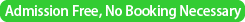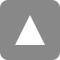﻿ 第24回統計的機械学習セミナー / The 24th Statistical Machine Learning Seminar | 統計数理研究所

# 第24回統計的機械学習セミナー / The 24th Statistical Machine Learning Seminar

Date&Time
2015年7月10日（金）13:30-
/ 10 July, 2015 (Fri) 13:30-Place

/ Seminar Room 2（D304）@ The Institute of Statistical MathematicsSpeaker
Dr. Ruriko Yoshida
(University of Kentucky; Visiting Assoc. Prof. at ISM)
Title
Extremal Positive Semidefinite Matrices for Weakly Bipartite Graphs
Abstract
For a graph $G$ with $p$ vertices the cone of concentration matrices consists of all real positive semidefinite $p\times p$ matrices with zeros in the off-diagonal entries corresponding to nonedges of~$G$.  The extremal rays of this cone and their associated ranks have applications to matrix completion problems, maximum likelihood estimation in Gaussian graphical models in statistics, and Gauss elimination for sparse matrices.  For a weakly bipartite graph $G$, we show that the normal vectors to the facets of the $(\pm1)$-cut polytope of $G$ specify the off-diagonal entries of extremal matrices in $\K_G$.  We also prove that the constant term of the linear equation of each facet-supporting hyperplane is the rank of its corresponding extremal matrix in $\K_G$. Furthermore, we show that if $G$ is series-parallel then this gives a complete characterization of all possible extremal ranks of $\K_G$, consequently solving the sparsity order problem for series-parallel graphs.
This is joint work with Liam Solus and Caroline Uhler.